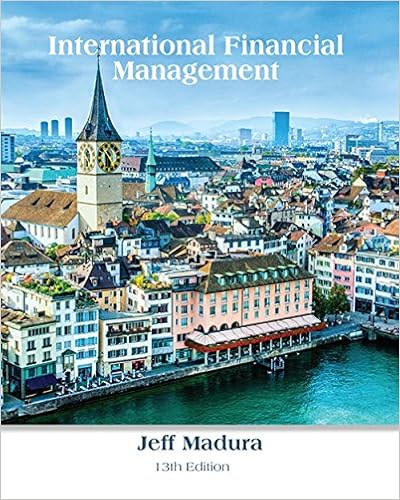# Find the value of a one year call option on 10000

• Notes
• khaehee
• 73
• 86% (182) 157 out of 182 people found this document helpful

This preview shows page 27 - 30 out of 73 pages.

##### We have textbook solutions for you!
The document you are viewing contains questions related to this textbook.The document you are viewing contains questions related to this textbook.
Chapter 5 / Exercise 29
International Financial ManagementExpert Verified
88. Find the value of a one-year call option on €10,000 with a strike price of $15,000. In one year the exchange rate (currently S 0 ($/€) = $1.50/€) can increase by 60% or decrease by 37.5% ( i.e. u = 1.6 and d = 0.625). The current one-year interest rate in the U.S. is i$ = 4% and the current one-year interest rate in the euro zone is i = 4% A. €1,525.52 B. $3,328.40 C.$4,992.60 D. €2,218.94 E. None of the above
89. Consider a 1-year call option written on £10,000 with an exercise price of $2.00 = £1.00. The current exchange rate is$2.00 = £1.00; The U.S. risk-free rate is 5% over the period and the U.K. risk-free rate is also 5%. In the next year, the pound will either double in dollar terms or fall by half (i.e. u = 2 and d = ½). If you write 1 call option, what is the value today (in dollars) of the hedge portfolio?
90. Value a 1-year call option written on £10,000 with an exercise price of $2.00 = £1.00. The spot exchange rate is$2.00 = £1.00; The U.S. risk-free rate is 5% and the U.K. risk-free rate is also 5%. In the next year, the pound will either double in dollar terms or fall by half (i.e. u = 2 and d = ½). Hint: H = .
7-27
##### We have textbook solutions for you!
The document you are viewing contains questions related to this textbook.The document you are viewing contains questions related to this textbook.
Chapter 5 / Exercise 29
International Financial ManagementExpert Verified
Chapter 07 Futures and Options on Foreign Exchange 91. Consider a 1-year call option written on £10,000 with an exercise price of $2.00 = £1.00. The current exchange rate is$2.00 = £1.00; The U.S. risk-free rate is 5% over the period and the U.K. risk-free rate is also 5%. In the next year, the pound will either double in dollar terms or fall by half (i.e. u = 2 and d = ½). If you write 1 call option and hedge it using an appropriate position in pounds what is your net cash flow (in dollars) at maturity if the exchange rate increases? Hint: H
92. Consider a 1-year call option written on £10,000 with an exercise price of $2.00 = £1.00. The spot exchange rate is$2.00 = £1.00; The U.S. risk-free rate is 5% and the U.K. risk-free rate is also 5%. In the next year, the pound will either double in dollar terms or fall by half (i.e. u = 2 and d = ½). If you write 1 call option and hedge it using an appropriate position in pounds, what is your net cash flow (in dollars) at maturity if the exchange rate increases? Hint: H = . A. £6,666.67 B. C. D. None of the above
•••